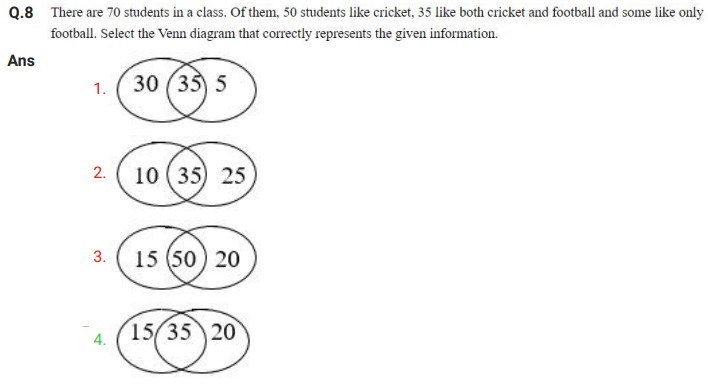# SSC CPO Exam Paper - 2019 "held on 13 December 2019" Shift-2 (Reasoning)## (Reasoning)

Q.1 Select the option in which the numbers share the same relationship as that shared by the given pair of numbers.
16 : 35
1. 43 : 67
2. 19 : 93
3. 14 : 35
4. 54 : 96

Q.2 If each of the letters of the English alphabet is assigned an odd numerical value beginning with A = 1, B = 3 and so on, what will be the total value of the letters of the word RADICAL?
1. 88
2. 89
3. 90
4. 99

Q.3 Select the option that is related to the third number in the same way as the second number is related to the first number.
91 : 104 :: 161 : ?
1. 235
2. 237
3. 184
4. 344

Q.4 Four numbers have been given, out of which three are alike in some manner and one is different. Select the number that is different from the rest.
1. 176
2. 160
3. 240
4. 178

Q.5 Four number-pairs have been given, out of which three are alike in some manner and one is different. Select the number-pair that is different from the rest.
1. 34 : 3
2. 17 : 6
3. 2 : 51
4. 24 : 4

Q.6 In the following number series, two numbers have been put within brackets. Select the most appropriate option for these numbers in relation to their inclusion in the series.
21, 24, 19, (29), 17, 28, (15), 30
1. The first bracketed number (from the left) is correct and the second bracketed number (from the left) is incorrect.
2. The first bracketed number (from the left) is incorrect and the second bracketed number (from the left) is correct.
3. Both the bracketed numbers are incorrect.
4. Both the bracketed numbers are correct.Q.9 Four number-pairs have been given, out of which three are alike in some manner and one is different. Select the number-pair that is different from the rest.
1. 7 : 37
2. 18 : 49
3. 13 : 29
4. 24 : 51